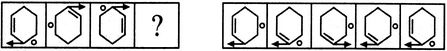Non Verbal Reasoning - Analogy - Discussion

Discussion :: Analogy - Section 1 (Q.No.16)

Each of the following questions consists of two sets of figures. Figures A, B, C and D constitute the Problem Set while figures 1, 2, 3, 4 and 5 constitute the Answer Set. There is a definite relationship between figures A and B. Establish a similar relationship between figures C and D by selecting a suitable figure from the Answer Set that would replace the question mark (?) in fig. (D).

16.

Select a suitable figure from the Answer Figures that would replace the question mark (?).

Problem Figures:                            Answer Figures:(A)     (B)      (C)     (D)                  (1)      (2)      (3)      (4)      (5)

 [A]. 1 [B]. 2 [C]. 3 [D]. 4 [E]. 5

Explanation:

The arrow moves four spaces (each space is equal to a side of the hexagon) in a CW direction while the line segment and the circle move two spaces in a CW direction.

 Vini said: (Jul 30, 2012) What about the circle ?

 Gitesh said: (Aug 4, 2017) Circle moves 2 sides clock-wise.

 Anand said: (Oct 13, 2018) @All. In B&C, the arrow is not moving at all. The explanation "The arrow moves four spaces (each space is equal to a side of the hexagon)" not seems to be correct.

 Honeiy said: (Sep 19, 2019) Agree @Anand. In B and C the arrow is not moving.